# 2D Arrays in JavaScript

Published on
76,530 Points
65,430 Views
16 Endorsements
Last Modified:
Awarded
Community Pick
This article shows how to create and access 2-dimensional arrays in JavaScript.  It includes a tutorial in case you are just trying to "get your head wrapped around" the concept and we'll also look at some useful tips for more advanced programmers.

## Introduction

A 2D array is a matrix of information.  Each element has two indexes: a row (y) and a column (x).   A matrix is handy whenever you have rows and columns of data.  For instance, if you need to output a month-by-month amortization table, you probably want to show it with a row for each year and a column for each month.  If you have a company sales chart, you might have a matrix with each regional branch represented as a row and have columns to break down sales for widgets, gadgets and gizmos.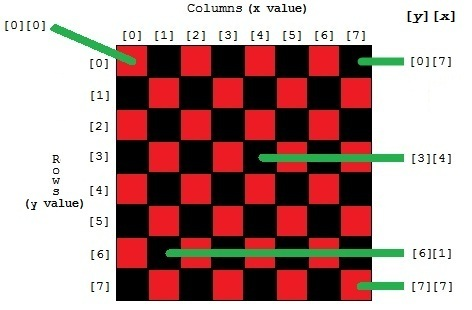Think of a chessboard.  There are 8 rows (numbered 0,1,...7) and 8 columns (also 0,1,...7). If you want to check what piece is on the top left corner, you access chessboard.  The next square to the right of that is , varying the x value until you get to  as you go left-to-right.  Vary the y value to move from the top to the bottom; for instance,  is the second square on the seventh row.

The notation I'm using matches that of JavaScript -- two sets of bracketed integers: [y][x]  All arrays start with element 0; the easy way to think of that is "how much distance from the left" a "distance of 0" means exactly at the left.

Another convention when talking about a matrix is the use of "generic" variable names x and y.  In such discussions, x is always the column index (distance from the left) and y is the row index (distance from the top).  So a y,x of  identifies the item at the top left.   is the second item on the top row, [n] is on the second row and so forth.

## JavaScript and 2D Arrays

Strictly speaking, JavaScript does not support 2D arrays.  The usual way to handle data in a 2D matrix is to create an Array object in which each element is, itself, an Array object.  We'll get to that in a minute.

Use a 1D Array As If It Were a 2D Array

It is possible to coerce a 1D array into a 2D array, using programming logic.  We'll touch on this lightly here.  It's interesting, but you might not need to use it in your day-to-day programming.

Let's say you have a string of letters:
``````var sData= "abcdefghijABCDEFGHIJäß©ÐëØ>½îÿ";
``````
You can see a pattern.  It appears to be three sequences of 10 characters each; that is, a 10x3 a matrix.
``````// column:  0123456789
var sData= "abcdefghij"  // row 0 (starts at offset 0)
+"ABCDEFGHIJ"  // row 1 (starts at offset 10)
+"äß©ÐëØ>½îÿ"  // row 2 (starts at offset 20)
``````
Since each row contains 10 columns, I can calculate the start of any row by multiplying the row index by 10.  In general, the formula is
(y * row_length) + x
where y is the row index, x is the column index, and row_length is the number of columns in each row.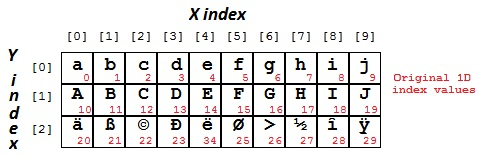Given a data string like that above, we can impose a 2D structure on it using program logic:
``````// assumes data is a string, sData and rows have 10 columns
function GetCellValue(y,x) {
nRowStart= y*10;
nOffset = nRowStart+ x;
sElementValue= sData.substr(nOffset,1); // access one element
return( sElementValue );
}
....
//                  y,x (row, column)
alert( GetCellValue(0,0) ); // displays a
alert( GetCellValue(0,1) ); // displays b
alert( GetCellValue(0,2) ); // displays c
alert( GetCellValue(1,2) ); // displays C
alert( GetCellValue(2,2) ); // displays ©
alert( GetCellValue(0,9) ); // displays j
alert( GetCellValue(1,9) ); // displays J
alert( GetCellValue(2,9) ); // displays ÿ
``````
With the above function, we can access the data string as a 2D array of individual characters.  All of that is to show that you can impose a 2D structure on a 1D array by adding a special data-access function.  But that's not the normal way to do things in JavaScript.

## Normal Usage: Array of Arrays

The normal way to use 2D arrays in JavaScript is to create a 1D array and set each element in it with another 1D array.

Straightforward Assignment

The following is one way to create and populate a 2D array:
``````as2D= new Array(); // an array of "whatever"
as2D= new Array("a","b","c","d","e","f","g","h","i","j" );
as2D= new Array("A","B","C","D","E","F","G","H","I","J" );
as2D= new Array("ä","ß","©","Ð","ë","Ø",">","½","î","ÿ" );
``````
That constructs and populates a three-element array; each element is a 10-element array of strings.  Now we can use normal JavaScript accessing syntax like:
``````alert( as2D ); // displays a
alert( as2D ); // displays ©
alert( as2D ); // displays ÿ
``````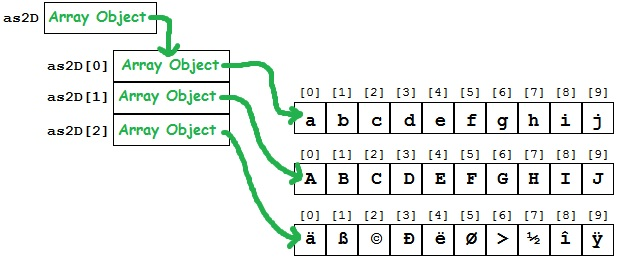Use Brackets [...] As a Shorthand

The syntax
var a= [ item0, item1, item2, ... ];
is shorthand for
var a= new Array( item0, item1, item2, ... );
That is,
[]            represents a new array with 0 elements,
["a"]       represents a new array with 1 string element,
["a","b"] represents a new array with 2 string elements,
and so forth.  Thus, a cool way to setup and populate our example array is:
``````var as2D = [
["a","b","c","d","e","f","g","h","i","j"],
["A","B","C","D","E","F","G","H","I","J"],
["ä","ß","©","Ð","ë","Ø",">","½","î","ÿ"]
];
``````
With that syntax, JavaScript constructs an Array-type variable that is exactly the same as with the
as2D[n]= new Array( a,b,c,... )
syntax that we used before.  You access the data the same way.

Use the Array.push() Method

The push() method of the Array object adds a new element (or elements) to the end.  Since it is common to populate an array starting from the top, you can use syntax like:
``````var as2D = new Array();
as2D = new Array();
as2D.push( "a" );
as2D.push( "b" );
as2D.push( "c","d","e","f","g","h","i" );
as2D.push( "j" );
as2D.push( new Array( "A","B","C","D","E","F","G","H","I","J" ) );
as2D.push( [ "ä","ß","©","Ð","ë","Ø",">","½","î","ÿ" ] );
``````
Once again, the above creates an Array of Array objects and it works the same as the earlier examples.

Note that the push() method lets you push a single item (as in lines 3, 4, and 6) or a series of items (line 5).  Lines 7 and 8 push entire arrays into the top-level array.  Here's how it looks without the extra illustrative lines:
``````var as2D= []; // or: new Array();
as2D.push( ["a","b","c","d","e","f","g","h","i","j"] );
as2D.push( ["A","B","C","D","E","F","G","H","I","J"] );
as2D.push( ["ä","ß","©","Ð","ë","Ø",">","½","î","ÿ"] );
``````
Use the String.split() Method

The split() method of the JavaScript String object returns an Array object.  It is very often used in populating an Array with predefined values.
``````var sData1= "a,b,c,d,e,f,g,h,i,j";
var sData2= "A,B,C,D,E,F,G,H,I,J";
var sData3= "ä,ß,©,Ð,ë,Ø,>,½,î,ÿ";

var as2D= []; // or: new Array();
as2D= sData1.split(",");
as2D= sData2.split(",");
as2D= sData3.split(",");
``````
The second parameter of the split() method identifies the delimiter character; in this case, we used a comma.  There is a useful idiom that will help us in this example: If the delimiter is the empty string (""), then the output is an array of individual characters.
``````var sData1= "abcdefghij";
var sData2= "ABCDEFGHIJ";
var sData3= "äß©ÐëØ>½îÿ";

var as2D= []; // or: new Array();
as2D= sData1.split("");
as2D= sData2.split("");
as2D= sData3.split("");
``````
...or...
``````var as2D= [];
as2D= "abcdefghij".split("");
as2D= "ABCDEFGHIJ".split("");
as2D= "äß©ÐëØ>½îÿ".split("");
``````
...or...
``````var as2D= "abcdefghij,ABCDEFGHIJ,äß©ÐëØ>½îÿ".split(",")
as2D=as2D.split("");
as2D=as2D.split("");
as2D=as2D.split("");
``````
...or even...
``````var as2D= [
"abcdefghij".split(""),
"ABCDEFGHIJ".split(""),
"äß©ÐëØ>½îÿ".split("")
];
``````
That last option kind of surprised me.  Coming from C++, I tend to expect that an array that is declared and populated in source code must be fully defined at compile time.  But JavaScript is different: that var declaration is just another piece of procedural code that gets executed at runtime.

## Processing a 2D Array

Nested for Loops

When you boil it all down, the main reason for creating and using a 2D array is so that at some point in your program, you can use a nested loop sequence like:
``````for ( var y=0; y<3; y++ ) {
for ( var x=0; x<10; x++ ) {
// do something with myArray[y][x]
}
}
``````
That is, step through each row and in each row step through each column to access the data at that row and column.  For instance, this code...
``````var sOut="<table border=2>";
for (var y=0; y<as2D.length; y++ ) {        // for each row
sOut += "<tr>";
for (var x=0; x<as2D[y].length; x++ ) { // for each clm
sOut += "<td>" + as2D[y][x] + "</td>";
}
sOut += "</tr>";
}
sOut += "</table>";
``````
... generates HTML that looks like this: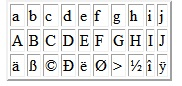And a little switcheroo of the columns and rows...
``````var nClmsPerRow= as2D.length;      // assume same length
for ( var x=0; x<nClmsPerRow; x++ ) {  // for each row
sOut += "<tr>";
for ( var y=0; y<as2D.length; y++ ) { // for each clm
sOut += "<td>" + as2D[y][x] + "</td>";
}
sOut += "</tr>";
}
``````
...and you can output a table as 10 rows of three columns.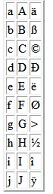Nested for...in loops

JavaScript also provides a specialized for-loop construct that iterates through an Array.  It is the for... in statement.  Using it simplifies the code a bit since it knows the loop-terminating condition (the end of the array).  It's used with collections and Arrays.  With Arrays, the syntax is:

for ( nIdxVar in aArray )

Each loop sets nIdxVar to the index of that iteration (0, 1, 2,...).  It stops looping when it gets to the end of the array.  Here's some code that accesses all items in our example 2D array:
``````for ( y in as2D ) {
for ( x in as2D[y] ) {
// do something with as2D[y][x];
}
}
``````
The real value of for...in comes when you have a sparse array ; that is, when some of the elements are undefined.  For instance:
``````var aSparse= new Array;
aSparse= ["zero",  "one",       "two"      ];
aSparse= [      ,  "forty-one",            ];
aSparse= ["fifty", "fifty-one", "fifty-two"];

for ( y in aSparse ) {
for ( x in aSparse[y] ) {
alert("y,x=(" +y+ "," +x+ ") value: " + aSparse[y][x] );
}
}
``````
...will skip rows 1-3, and the columns 0 and 2 of row 4 -- the elements that have not been populated.  It will output:
y,x=(0,0) value: zero
y,x=(0,1) value: one
y,x=(0,2) value: two
y,x=(4,1) value: forty-one
y,x=(5,0) value: fifty
y,x=(5,1) value: fifty-one
y,x=(5,2) value: fifty-two

## 2D Array vs. Collection of Objects

A 2D array is great for representing a matrix of identical data elements.  For instance, a chessboard is an 8x8 matrix of squares, and a screen is a 1680x1050 matrix of pixels.  Such x-by-y matrices come into use often in advanced JavaScript programming.

But an even more common scenario is a 1D array that is a list of groups of different items.  That includes such often seen constructs as a database recordset, lists of <input> objects on a webpage, data related to items in a shopping cart, and so forth.

In my next installment, well look at some fundamental aspects of such collections of data and how to create and use them in JavaScript.  See:

Maps and Collections in JavaScript
and
Sorting Arrays and Collections in JavaScript
=-=-=-=-=-=-=-=-=-=-=-=-=-=-=-=-=-=-=-=-=-=-=-=-=-=-=-=-=-=-=-=-=-=-=-=-=-=-=-=-=-=
If you liked this article and want to see more from this author, please click the Yes button near the:
Was this article helpful?
label that is just below and to the right of this text.   Thanks!
=-=-=-=-=-=-=-=-=-=-=-=-=-=-=-=-=-=-=-=-=-=-=-=-=-=-=-=-=-=-=-=-=-=-=-=-=-=-=-=-=-=
16
Ask questions about what you read
If you have a question about something within an article, you can receive help directly from the article author. Experts Exchange article authors are available to answer questions and further the discussion.
Get 7 days free